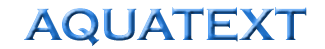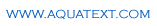The Free On-line Aquaculture DictionaryCompressor / blower - Power equations
 P = (Q x H) / (6.1 x n) Where P = Power (kW) Q = Air flow (m3 / minute) H = System head (m) = sum of resistance head = suction head  + pipe losses + device losses (eg diffuser) + depth of water n = Efficiency of compressor / blower typically 60% - 70%